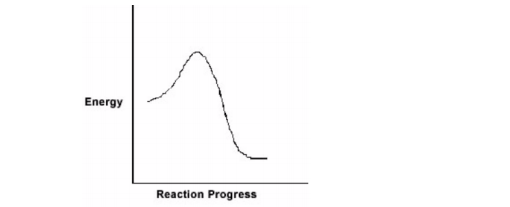# Problem: For the chemical reaction system described by the diagram below which statement is true?  If the Ea for the forward reaction is 25 kJ/mol and the enthalpy of reaction is -95 kJ/mol, what is Ea for the reverse reaction? A. 120 kJ/mol B. 70 kJ/mol C. 95 kJ/mol D. 25 kJ/mol E. -70 kJ/mol

###### FREE Expert Solution
97% (140 ratings)
###### Problem Details

For the chemical reaction system described by the diagram below which statement is true?

If the Ea for the forward reaction is 25 kJ/mol and the enthalpy of reaction is -95 kJ/mol, what is Ea for the reverse reaction?

A. 120 kJ/mol

B. 70 kJ/mol

C. 95 kJ/mol

D. 25 kJ/mol

E. -70 kJ/molWhat scientific concept do you need to know in order to solve this problem?

Our tutors have indicated that to solve this problem you will need to apply the Energy Diagram concept. If you need more Energy Diagram practice, you can also practice Energy Diagram practice problems.

What is the difficulty of this problem?

Our tutors rated the difficulty ofFor the chemical reaction system described by the diagram be...as medium difficulty.

How long does this problem take to solve?

Our expert Chemistry tutor, Jules took 1 minute and 16 seconds to solve this problem. You can follow their steps in the video explanation above.

What professor is this problem relevant for?

Based on our data, we think this problem is relevant for Professor Keirstead's class at UNE.